Constructing a triangle when 2 angles and side between is known (ASA)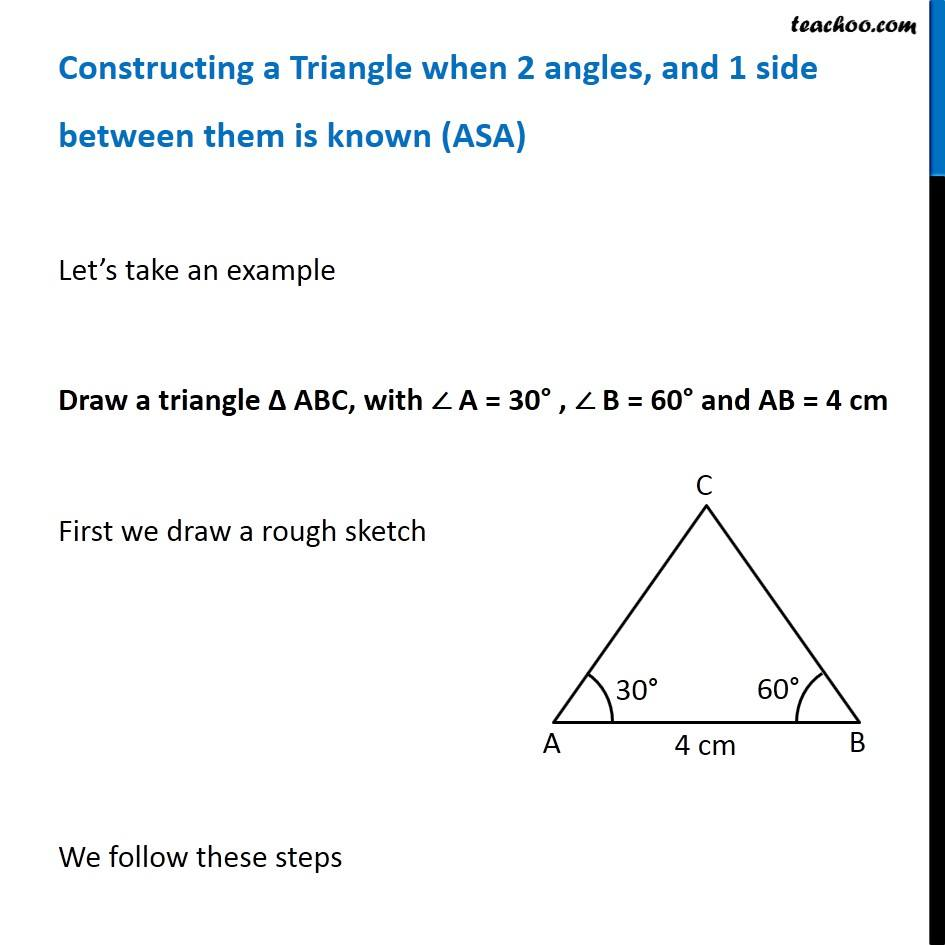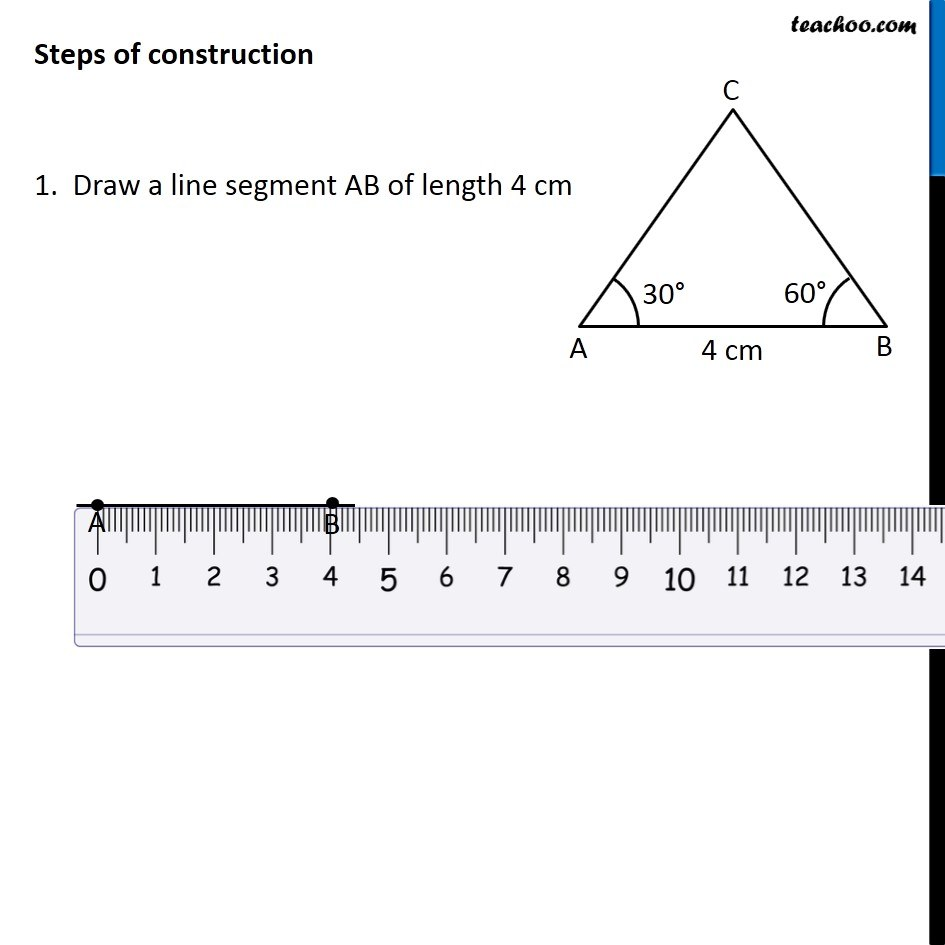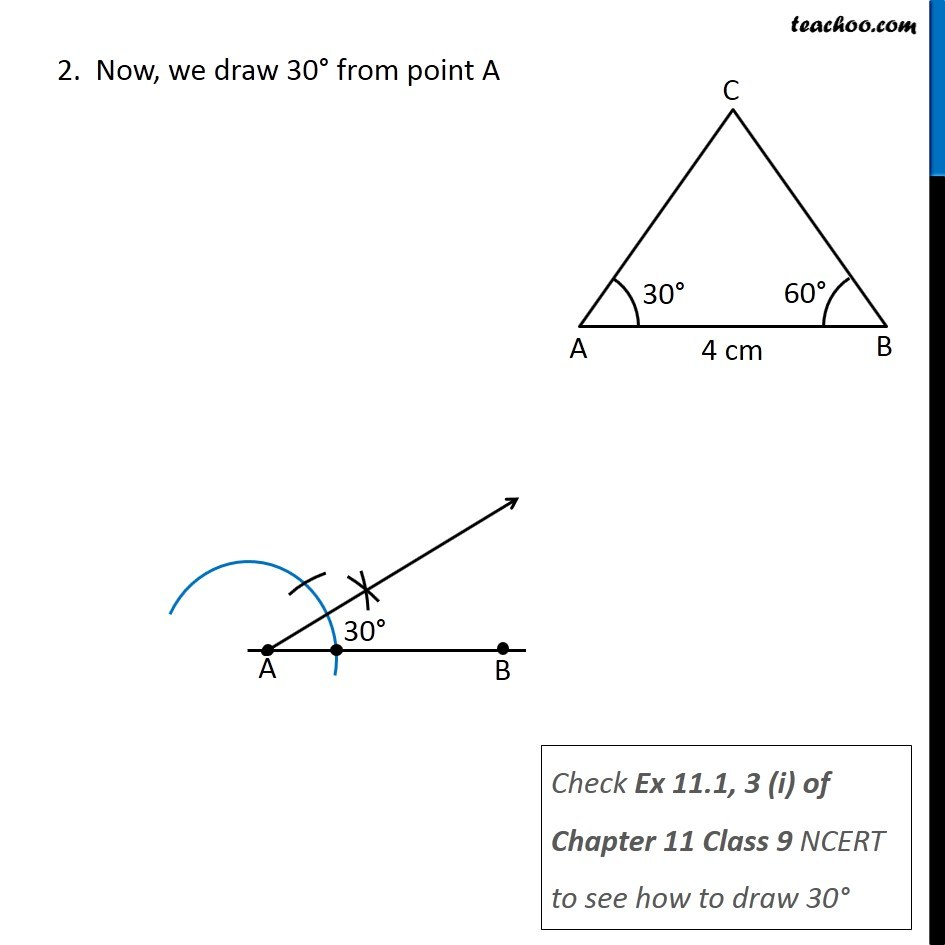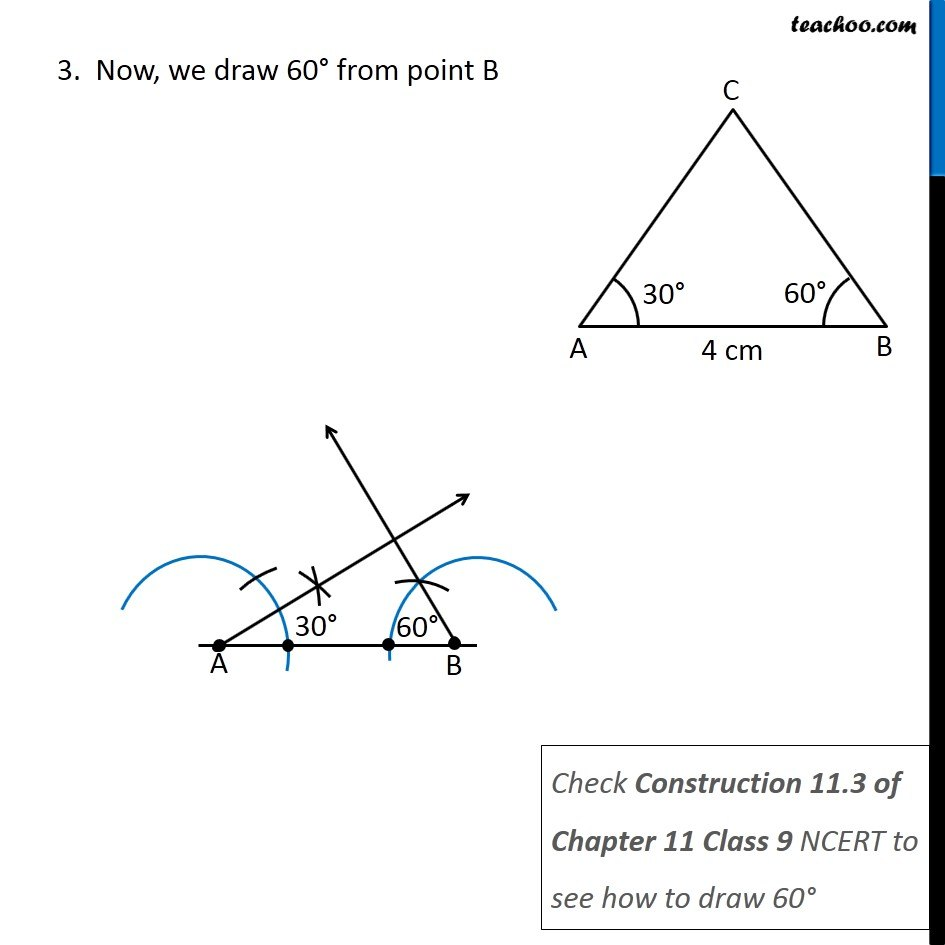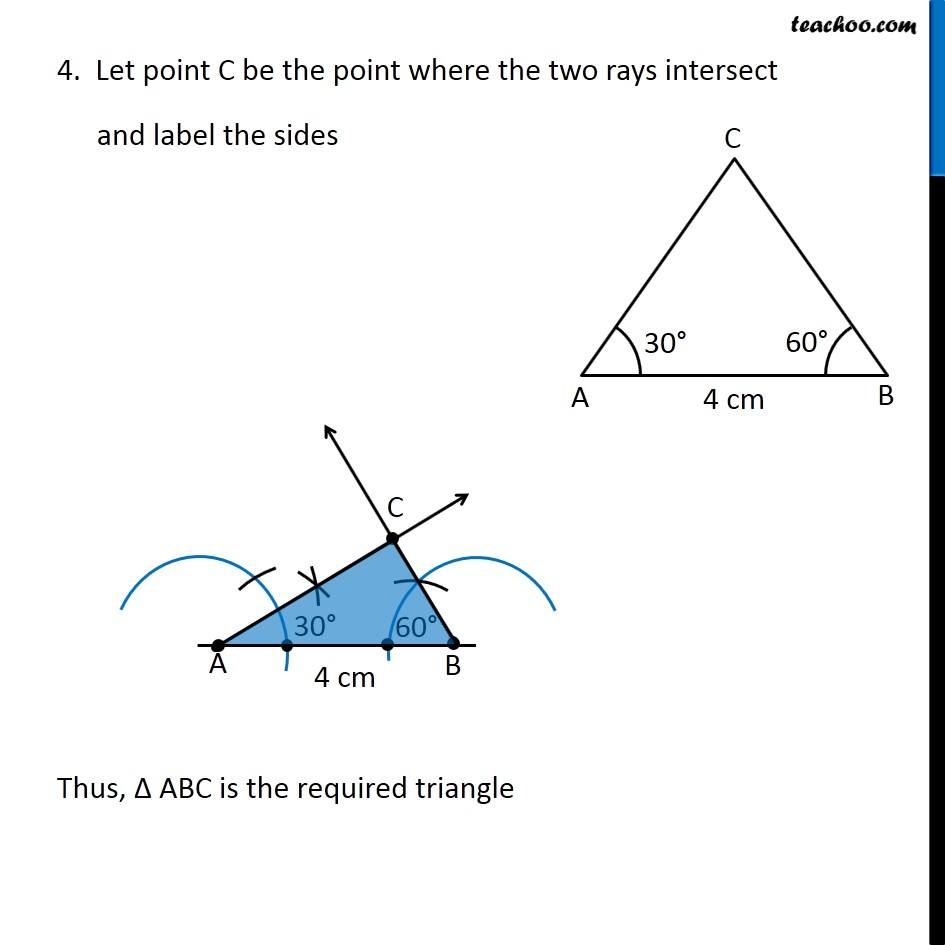Learn in your speed, with individual attention - Teachoo Maths 1-on-1 Class

### Transcript

Constructing a Triangle when 2 angles, and 1 side between them is known (ASA) Let’s take an example Draw a triangle Δ ABC, with ∠ A = 30° , ∠ B = 60° and AB = 4 cm First we draw a rough sketch We follow these steps Steps of construction 1. Draw a line segment AB of length 4 cm 2. Now, we draw 30° from point A Check Ex 11.1, 3 (i) of Chapter 11 Class 9 NCERT to see how to draw 30° 3. Now, we draw 60° from point B Check Construction 11.3 of Chapter 11 Class 9 NCERT to see how to draw 60° 4. Let point C be the point where the two rays intersect and label the sides Thus, Δ ABC is the required triangle